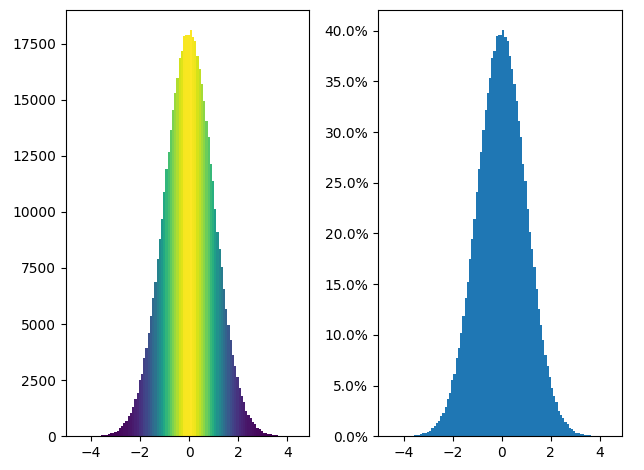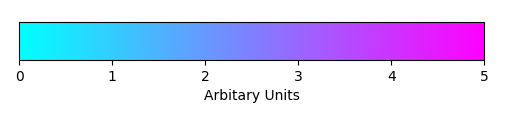# Matplotlib.colors.Normalize class in Python

Matplotlib is an amazing visualization library in Python for 2D plots of arrays. Matplotlib is a multi-platform data visualization library built on NumPy arrays and designed to work with the broader SciPy stack.

## matplotlib.colors.Normalize

The matplotlib.colors.Normalize class belongs to the matplotlib.colors module. The matplotlib.colors module is used for converting color or numbers arguments to RGBA or RGB.This module is used for mapping numbers to colors or color specification conversion in a 1-D array of colors also known as colormap.

The matplotlib.colors.Normalize class is used to normalize data into the interval of [0.0, 1.0].

Syntax:

class matplotlib.colors.Normalize(vmin=None, vmax=None, clip=False)

If either of vmin or vmax is not set then it initializes from the minimum and maximum value of the first input processed respectively. In other words, __call__(Data) calls autoscale_None(Data). If the value of clip is set to True and the given value is out of range then it returns 0 or 1, whichever is the closest. If vmax==vmin then it returns 0. It operates with both scalar or arrays including masked arrays. The masked values are set to 1 if clip is True, else they remain masked.

Methods:

1. autoscale(self, A): This method sets the vmin to min and vmax to max of A.
2. autoscale_None(self, A): This method autoscales only vmin and vmax with None value.
3. inverse(self, value): It interchanges the values of vmin and vmax.
4. static process_value(value): The value parameter in this method can be a scalar or a sequence. It is used to homogenize input values for efficient and simple normalization. This method returns a masked array of matching values. All float data types are preserved and integer data types with two or smaller bytes are transformed to np.float32, while the larger bytes type are transformed into np.float64. This is done to improve speed for larger arrays by preserving float32 values whenever possible through using in-place operations.
5. scaled(self): It returns a boolean to check if vmin or vmax is set.

Example 1:

 `import` `matplotlib.pyplot as plt ` `import` `numpy as np ` `from` `matplotlib ``import` `colors ` `from` `matplotlib.ticker ``import` `PercentFormatter ` ` `  `# set a  random state for  ` `# reproducibility ` `np.random.seed(``19687581``) ` ` `  `total_points ``=` `500000` `total_bins ``=` `100` ` `  `# Centering at a = 0 and b = 5 ` `# generate normal distributions ` `a ``=` `np.random.randn(total_points) ` `b ``=` `.``4` `*` `a ``+` `np.random.randn(``500000``) ``+` `5` ` `  `figure, axes ``=` `plt.subplots(``1``, ``2``,  ` `                            ``tight_layout ``=` `True``) ` ` `  `# C is the count in each bin ` `C, bins, patches ``=` `axes[``0``].hist(a, ` `                                ``bins ``=` `total_bins) ` ` `  `# We'll color code by height, ` `# but you could use any scalar ` `fracs ``=` `C ``/` `C.``max``() ` ` `  `# Normalize of  the data to 0..1  ` `# for covering the full range of  ` `# the colormap ` `norm ``=` `colors.Normalize(fracs.``min``(), fracs.``max``()) ` ` `  `# looping through the objects and  ` `# setting the color of each accordingly ` `for` `thisfrac, thispatch ``in` `zip``(fracs, patches): ` `    ``color ``=` `plt.cm.viridis(norm(thisfrac)) ` `    ``thispatch.set_facecolor(color) ` ` `  `# normalize the inputs by C ` `axes[``1``].hist(a, bins ``=` `total_bins, density ``=` `True``) ` ` `  `# formating the y-axis for displaying ` `# percentage ` `axes[``1``].yaxis.set_major_formatter(PercentFormatter(xmax ``=` `1``)) `

Output:Example 2:

 `import` `matplotlib.pyplot as plt ` `import` `matplotlib as mpl ` ` `  `figure, axes ``=` `plt.subplots(figsize ``=``(``6``, ``1``)) ` `figure.subplots_adjust(bottom ``=` `0.5``) ` ` `  `color_map ``=` `mpl.cm.cool ` `normlizer ``=` `mpl.colors.Normalize(vmin ``=` `0``, vmax ``=` `5``) ` ` `  `figure.colorbar(mpl.cm.ScalarMappable(norm ``=` `normlizer, ` `               ``cmap ``=` `color_map), ` `               ``cax ``=` `axes, orientation ``=``'horizontal'``, ` `               ``label ``=``'Arbitary Units'``) `

Output:My Personal Notes arrow_drop_upCheck out this Author's contributed articles.

If you like GeeksforGeeks and would like to contribute, you can also write an article using contribute.geeksforgeeks.org or mail your article to contribute@geeksforgeeks.org. See your article appearing on the GeeksforGeeks main page and help other Geeks.

Please Improve this article if you find anything incorrect by clicking on the "Improve Article" button below.

Article Tags :

Be the First to upvote.

Please write to us at contribute@geeksforgeeks.org to report any issue with the above content.